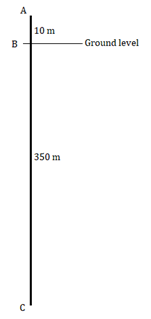# An elevator descends into a mine shaft at the rate of 6 m/min. The descent starts from 10 m above the ground level. How long will it take to reach $-$350 m?

Given:

An elevator descends into a mine shaft at the rate of 6 m/min.

The descent starts from 10 m above the ground level.

To find: Here we have to find the time taken by the elevator to reach $-$350 m.

Solution:In the above figure, A represents the current position of the elevator which is 10 m above ground level. B is the ground level. And point C is 350 m below the ground level.

Now,

Total distance $=$ AB $+$ BC

Total distance $=$ 10 $+$ 350 m

Total distance $=$ 360 m

Given that speed of elevator $=$ 6 m/min

So,

$Time\ =\ \frac{Distance}{Speed}$

$Time\ =\ \frac{360}{6} \ \ minutes$

$\mathbf{Time\ =\ 60\ \ minutes}$

or,

$\mathbf{Time\ =\ 1\ hour}$

Therefore, the elevator will take 1 hour to reach its destination.

Updated on: 10-Oct-2022

41 Views